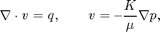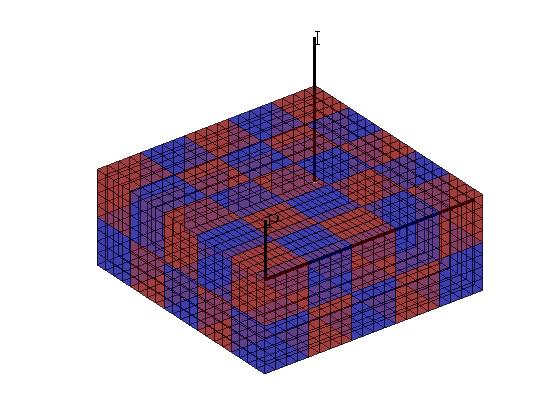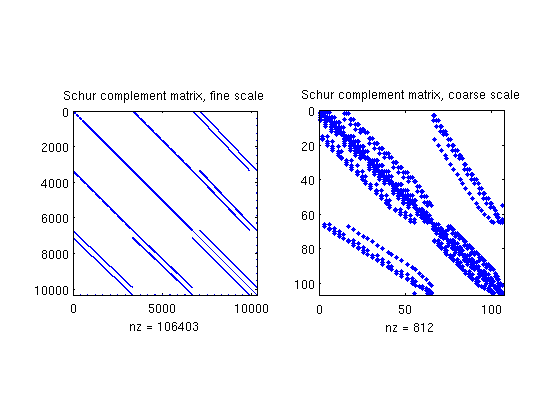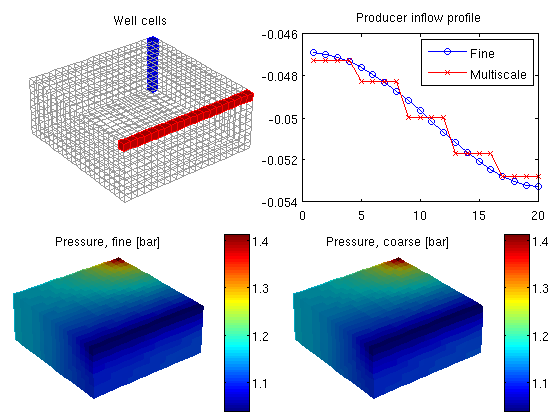#Multiscale Flow Solver: I

Compare the fine-grid and the multiscale pressure solver by solving the single-phase pressure equationfor a Cartesian grid with isotropic, homogeneous permeability. This example is built upon the flow-solver tutorial "Using Peacemann well models".

## Define the model and set data

We construct the Cartesian grid, set the permeability to 100 mD, and use the default single-phase fluid with density 1000 kg/m^3 and viscosity 1 cP.

nx = 20; ny = 20; nz = 8;
Nx =  5; Ny =  5; Nz = 2;
verbose   = false;
G         = cartGrid([nx ny nz]);
G         = computeGeometry(G);
rock.perm = repmat(100*milli*darcy, [G.cells.num, 1]);
fluid     = initSingleFluid('mu' ,    1*centi*poise     , ...
'rho', 1000*kilogram/meter^3);


Set two wells, one vertical and one horizontal. Note that a vertical well can be constructed by both addWell and verticalWell, so the appropriate choice depends on whether you know the well cells or the I, J, K position of the well.

W = verticalWell([], G, rock, nx, ny, 1:nz,            ...
'Type', 'rate', 'Val', 1*meter^3/day, ...
'Radius', .1, 'Name', 'I', 'Comp_i', [1, 0]);
W = addWell(W, G, rock, 1:nx, 'Type','bhp', ...
'Val', 1*barsa, 'Radius', .1, 'Dir', 'x', ...
'Name', 'P', 'Comp_i', [0, 1]);


## Set up solution structures

Here we need four solution structures, two for each simulator to hold the solutions on the grid and in the wells, respectively.

xRef = initState(G, W, 0, [0, 1]);
xMs  = xRef;


## Partition the grid

We partition the fine grid into a regular Nx-by-Ny-by-Nz coarse grid in index space so that each coarse block holds (nx/Nx)-by-(ny/Ny)-by-(nz/Nz) fine cells. The resulting vector p has one entry per fine-grid cell giving the index of the corresponding coarse block. After the grid is partitioned in index space, we postprocess it to make sure that all blocks consist of a connected set of fine cells. This step is superfluous for Cartesian grids, but is required for grids that are only logically Cartesian (e.g., corner-point and other mapped grids that may contain inactive or degenerate cells).

p = partitionUI(G, [Nx, Ny, Nz]);
p = processPartition(G, p, 'Verbose', verbose);

% Generate the coarse-grid structure
CG = generateCoarseGrid(G, p, 'Verbose', verbose);


Plot the partition and the well placement

clf
plotCellData(G, mod(p,2),'EdgeColor','k','FaceAlpha',0.5,'EdgeAlpha', 0.5);
plotWell(G, W, 'radius', 0.1, 'color', 'k');
view(3), axis equal tight off## Assemble linear systems

First we compute the inner product to be used in the fine-scale and coarse-scale linear systems. Then we generate the coarse-scale system

gravity off
S  = computeMimeticIP(G, rock, 'Verbose', verbose);

mu  = fluid.properties(xMs);
kr  = fluid.relperm(ones([G.cells.num, 1]), xMs);
mob = kr ./ mu;
CS  = generateCoarseSystem(G, rock, S, CG, mob, 'Verbose', verbose);


Then, we assemble the well systems for the fine and the coarse scale.

W = generateCoarseWellSystem(G, S, CG, CS, mob, rock, W);
disp('W(1):'); display(W(1));
disp('W(2):'); display(W(2));

W(1):
cells: [8x1 double]
type: 'rate'
val: 1.1574e-05
r: 0.1000
dir: [8x1 char]
WI: [8x1 double]
dZ: [8x1 double]
name: 'i'
compi: [1 0]
refDepth: 0
sign: 1
CS: [1x1 struct]

W(2):
cells: [20x1 double]
type: 'bhp'
val: 100000
r: 0.1000
dir: [20x1 char]
WI: [20x1 double]
dZ: [20x1 double]
name: 'p'
compi: [0 1]
refDepth: 0
sign: []
CS: [1x1 struct]



## Solve the global flow problems

xRef = solveIncompFlow  (xRef, G, S, fluid, 'wells', W, ...
'Solver', S.type, 'MatrixOutput', true);
xMs  = solveIncompFlowMS(xMs , G, CG, p, S, CS, fluid, 'wells', W, ...
'Solver', S.type, 'MatrixOutput', true);

% Report pressure in wells.
dp = @(x) num2str(convertTo(x.wellSol(1).pressure, barsa));
disp(['DeltaP, Fine: ', dp(xRef)])
disp(['DeltaP, Ms:   ', dp(xMs )])

DeltaP, Fine: 1.2093
DeltaP, Ms:   1.2115


## Plot Schur complement matrices

clf
subplot(1,2,1); spy(xRef.A); title('Schur complement matrix, fine scale');
subplot(1,2,2); spy(xMs.A);  title('Schur complement matrix, coarse scale');## Plot solution

clf
subplot('Position', [0.02 0.52 0.46 0.42]),
plotGrid(G, 'FaceColor', 'none', 'EdgeColor', [.6, .6, .6]);
plotGrid(G, W(1).cells, 'FaceColor', 'b', 'EdgeColor', 'b');
plotGrid(G, W(2).cells, 'FaceColor', 'r', 'EdgeColor', 'r');
title('Well cells')
view(3), camproj perspective, axis tight equal off, camlight headlight

subplot('Position', [0.54 0.52 0.42 0.40]),
plot(convertTo(xRef.wellSol(2).flux, meter^3/day), '-ob'); hold on
plot(convertTo(xMs .wellSol(2).flux, meter^3/day), '-xr');
legend('Fine', 'Multiscale')
title('Producer inflow profile')

subplot('Position', [0.02 0.02 0.46 0.42]),
plotCellData(G, convertTo(xRef.pressure(1:G.cells.num), barsa))
title('Pressure, fine [bar]')
view(3), camproj perspective, axis tight equal off, camlight headlight
cax = caxis; colorbar

subplot('Position', [0.52 0.02 0.46 0.42]),
plotCellData(G, convertTo(xMs.pressure(1:G.cells.num), barsa));
title('Pressure, coarse [bar]')
view(3), camproj perspective, axis tight equal off, camlight headlight
caxis(cax); colorbarPublished March 4, 2011